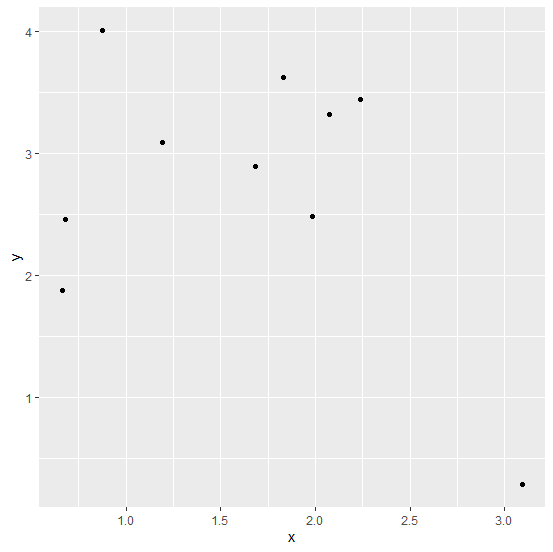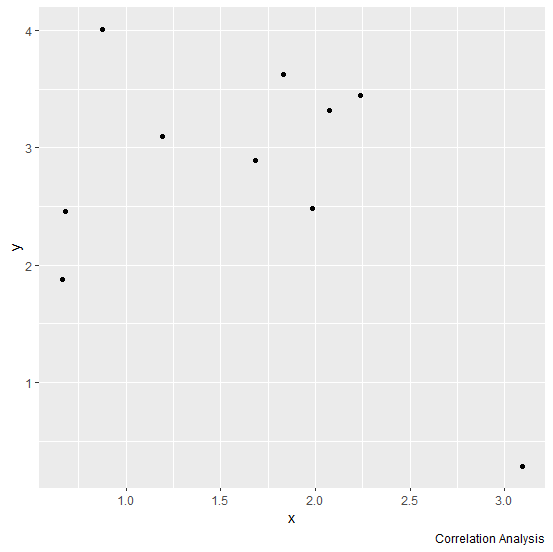# How to add a citation in a plot created by using ggplot2 in R?

A footnote is generally used to give references to a document, text or image and it is called citation. It helps the reader or viewer to check out the original source using the new text or image is generated. If we want to give citation to a plot in R using ggplot2 package then we can add labs that has caption option to add the footnotes.

## Example

Consider the below data frame −

Live Demo

> set.seed(1)
> x<-rnorm(10,1.5)
> y<-rnorm(10,2.5)
> df<-data.frame(x,y)
> df

## Output

      x       y
1 0.8735462 4.0117812
2 1.6836433 2.8898432
3 0.6643714 1.8787594
4 3.0952808 0.2853001
5 1.8295078 3.6249309
6 0.6795316 2.4550664
7 1.9874291 2.4838097
8 2.2383247 3.4438362
9 2.0757814 3.3212212
10 1.1946116 3.0939013

Loading ggplot2 package and creating a scatterplot between x and y −

> library(ggplot2)
> ggplot(df,aes(x,y))+geom_point()

## OutputCreating the plot with footnote −

> ggplot(df,aes(x,y))+geom_point()+labs(caption="Correlation Analysis")

## Output